##### Traveling Wave Solutions for a Coupled KdV Equations

Ye Zhang, Shengqiang Tang

Journal of Advances in Mathematics and Computer Science, Page 1027-1034
DOI: 10.9734/BJMCS/2014/5474

In this paper, we use a method in order to find exact explicit traveling solutions in the subspace of the phase space for coupled KdV equations. The key idea is removing a coupled relation for the given system so that the new systems can be solved. The existence of solitary wave solutions is obtained. It is shown that bifurcation theory of dynamical systems provides a powerful mathematical tool for solving a great many nonlinear partial differential equations in mathematical physics.

##### Multistage HPM Applied to Path Tracking Damped Oscillations of a Model for HIV Infection of CD4+ T Cells

H. Vazquez-Leal, L. Hernandez-Martinez, Y. Khan, V.M. Jimenez-Fernandez, U. Filobello-Nino, A. Diaz-Sanchez, A. L. Herrera-May, R. Castaneda-Sheissa, A. Marin-Hernandez, F. Rabago-Bernal, J. Huerta-Chua

Journal of Advances in Mathematics and Computer Science, Page 1035-1047
DOI: 10.9734/BJMCS/2014/7714

The behavior of CD4+ T cells infection is modeled by a differential equation system with no exact analytical solution. This work proposes the application of Multistage Homotopy Perturbation Method (MuHPM) to track the path of damped oscillations in the evolution of HIV infection in CD4+ T cells. In addition, the paper presents the results which compare the following methods: MuHPM, Modied Variational Iteration Method (MVIM) and HPM, and showing that MuHPM analytical-numerical method is more precise than the other methods, reaching to an adequately plot 70 days of the progress of infection instead of the 0.8 days and 2 days attained by HPM and MVIM methods respectively.

##### General Equilibria

Vasile Postolică

Journal of Advances in Mathematics and Computer Science, Page 1048-1073
DOI: 10.9734/BJMCS/2014/5967

This research work is devoted to general equilibria in abstract spaces, through the agency of the ideal points, fixed points of multifunctions, Isac’s cones, Choquet boundaries and applied mathematics for academic and industrialists engaged in eco - efficiency. It can be also considered as a short survey of the recent results obtained in this field.

##### Applications of Liouville’s Identity with an Odd Function

Aeran Kim, Keum Yeon Lee, Hwasin Park

Journal of Advances in Mathematics and Computer Science, Page 1074-1090
DOI: 10.9734/BJMCS/2014/7957

In this paper, we are based on the Huard, Ou, Spearman and Williams’s generalization of Liouville’s

Identity so we obtain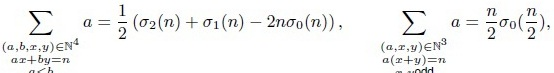and etc. Also, independently we attempt to consider the Liouville’s Identity, therefore as the application of his identity, we have the restricted combinatoric convolution sums as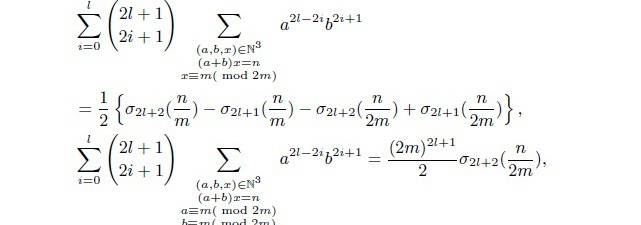(see Theorem 1.6 and Theorem 1.8) and etc., by dealing with an odd function for m, n ∈and l ∈∪ {0}.

##### Weighted Hesitant Fuzzy Sets and Their Application to Multi-Criteria Decision Making

Zhiming Zhang, Chong Wu

Journal of Advances in Mathematics and Computer Science, Page 1091-1123
DOI: 10.9734/BJMCS/2014/8533

Aims: The aim of this paper is to investigate weighted hesitant fuzzy sets and their application to multi-criteria decision making.
Study Design: This paper puts forward the concept of a weighted hesitant fuzzy set (WHFS), in which several possible membership degrees of each element have different weights. Archimedean t-conorm and t-norm provide a generalization of a variety of other t-conorms and t-norms that include as special cases Algebraic, Einstein, Hamacher and Frank t-conorms and t-norms.
Place and Duration of Study: Hesitant fuzzy set, permitting the membership degree of an element to be a set of several possible values, can be referred to as an efficient mathematical tool for modeling people’s hesitancy in daily life. It is noted that several possible membership degrees of each element in the hesitant fuzzy set are of equal importance, but in many practical problems, especially in multi-criteria decision making, the weights of several possible membership degrees of each element should be taken into account.
Methodology: In this paper, based on Archimedean t-conorm and t-norm, we present some operations on weighted hesitant fuzzy sets (WHESs), and based on which, we develop two weighted hesitant fuzzy aggregation operators for aggregating weighted hesitant fuzzy information. Furthermore, some desired properties and special cases of the developed operators are discussed in detail.
Results: We develop an approach for multi-criteria decision making under weighted hesitant fuzzy environment.
Conclusion: An illustrative example is provided to show the effectiveness and practicality of the proposed operators and approach.

##### Generalized Sturm-Liouville Problems and Chebychev Collocation Method

Mohamed El-Gamel, Mona Sameeh

Journal of Advances in Mathematics and Computer Science, Page 1124-1133
DOI: 10.9734/BJMCS/2014/7670

In this paper, we present an algorithm for approximating the eigenvalues of Sturm-Liouville problems with parameter-dependent boundary conditions. The algorithm is based on the Chebychev method. A few examples shall be presented to illustrate the proposed method and a comparison made with the regularized sampling method. It is shown that the Chebychev method yields better results.

##### Generalizing the Asymmetric Run-length-limited Systems

D. Ahmadi Dastjerdi, S. Jangjooye Shaldehi

Journal of Advances in Mathematics and Computer Science, Page 1134-1145
DOI: 10.9734/BJMCS/2014/7434

For i = 1; 2, if Xi is a synchronized system generated by Vi = {ui I : ∝I ui  ∝I  ∈ B (Xi), ∝I  ui }  where _i is a synchronizing word for Xi, then a natural generalization of an asymmetric-RLL(d1; k1; d0; k0) systems is a coded system Z generated by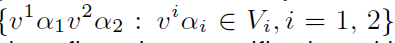We investigate the dynamical properties of Z. We show that Z is sofic or has specification with variable gap length (SVGL) if and only if X1 and X2 are so. Also, if Z is SFT or AFT, then X and Y are SFT or AFT respectively and sufficient conditions for the converse will be given.

##### On the Superstability of Trigonometric Type Functional Equations

D. Zeglami, S. Kabbaj

Journal of Advances in Mathematics and Computer Science, Page 1146-1155
DOI: 10.9734/BJMCS/2014/7792

The aim of this paper is to study the superstability for the mixed trigonometric functional equation: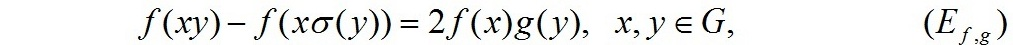and the stability of the Pexider type functional equation: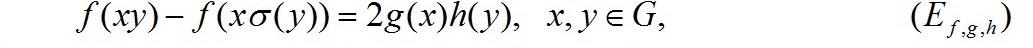where  G is any group, not necessarily abelian  f , g and  h are unknown complex valued functions and σ is an involution of G.   As a consequence we prove that if f satisfies the inequality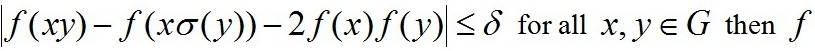for all x , y G then  f is bounded.

##### New Bounds for Restricted Isometry Constant for the s-sparse Recovery via Compressed Sensing

Hiroshi Inoue

Journal of Advances in Mathematics and Computer Science, Page 1156-1165
DOI: 10.9734/BJMCS/2014/9002

The main purpose of this paper is to establish the sufficient condition for the restricted isometry constant δs in compressed sensing by using T. Cai and A. Zhang idea. Let h ≡ x* − x and h = (h1; h2; _ _ _ ; hn), where x is an unknown signal and x* is the CS-solution. For simplicity, we assume that the index of h is sorted by| h1 |≥ | h2| ≥  ….. ≥  |hn|. Let s be a fixed positive integer, T0 = {1; 2,…., s} and T1 ⊂ T0. In this paper, we focus the quality of hT0 and research good conditions for the recovery of sparse signals by investigating the difference between||hT1||1 and ||hTf||1. We shall show that if δs < 0:5 under an assumption for ||hT1||1, and similarly if δ 34 s < 0:414 or δ 24 25 s < 0:436, then we have stable recovery of approximately sparse signals.

##### On Perfect Commutative EIFA-rings

Mohameth Alassane Ndiaye, Cheikh Thiécoumba Gueye

Journal of Advances in Mathematics and Computer Science, Page 1166-1169
DOI: 10.9734/BJMCS/2014/7863

We consider the class F of endo-Artinian modules, i.e. the modules M which satisfying the descending chain condition for endomorphic images: any descending chain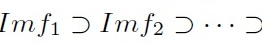is stationary, where. Let A be the class of Artinian modules. It is clear that every Artinian R-moduleM is endo-Artinian, so A ⊂  F, but the converse is not true. Indeed,is a non-Artinian-module which is endo-Artinian. The aim of this work, is to characterize perfect commutative rings for which F and A are identical.